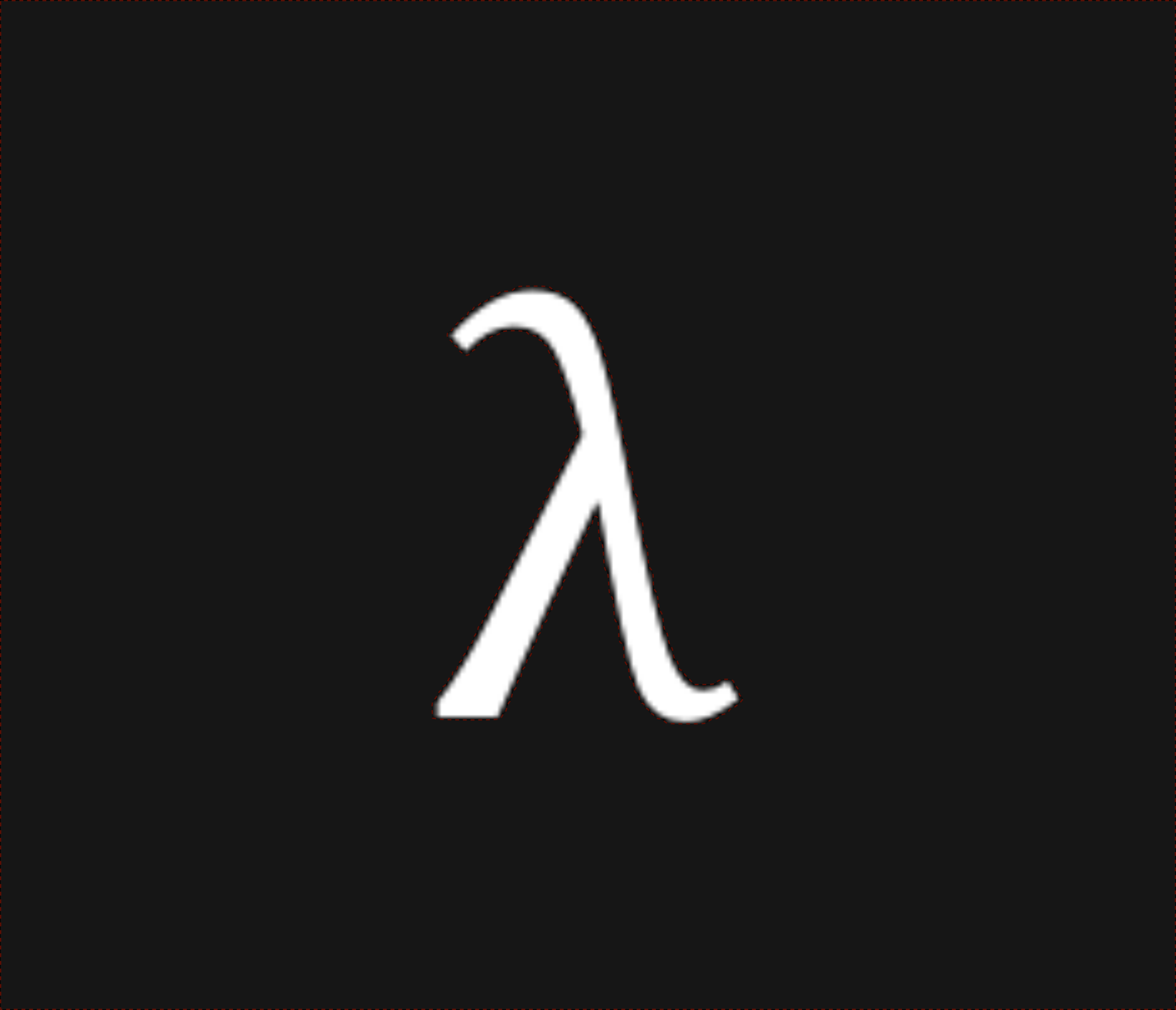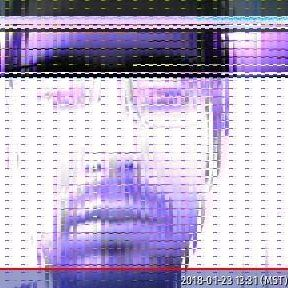nixpulvis

## Achilles' Tent Notes #12 written by Nathan Lilienthal on December 02, 2019.

### Graph Notation

To run an MPC we must be able to label each party (and virtual party) which constitutes the protocol. Based on the architectures given as challenge problems in the HECTOR GFI, we attempt to create a notation for easily describing these topologies.

#### Fully Connected Graphs

Sets of fully connected parties are denoted with {}, for example the following shows a simple network of four parties:

G = { A, B, C, D }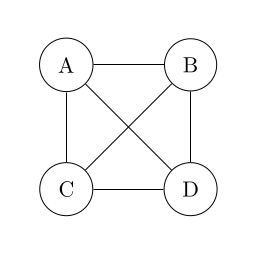We can nest sets within each other:

G = { { A, B }, { C, D } }


The two examples above are equivalent, however it may be useful to label specific groups of nodes anyway.

H = { A, B }
I = { C, D }
G = { Q, R }


#### Fully Disconnected Graphs

We can also define fully disconnected graphs with [].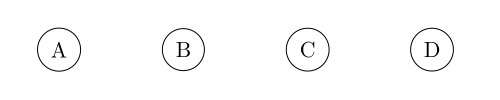G = [A, B, C, D]


While a disconnected graph may seem useless by itself, when nested within a larger connected graph they allow us to represent arbitrarily complex topologies of graphs (TODO: is this actually true?).

#### Star Graphs

For example a “star” network with four edge nodes and a single center node can be represented like this: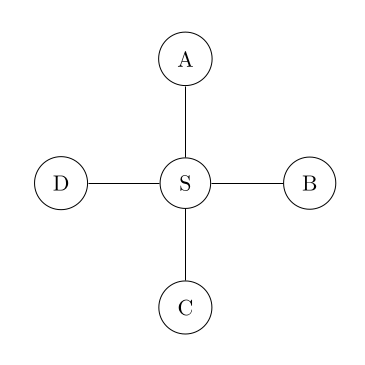G = { S, [A, B, C, D] }
= [{ S, A }, { S, B }, { S, C }, { S, D }]


Or a more complex example of a “server star” graph, linked into an MPC protocol.

I = [I1, I2, I3, I4, I5]
S = {S1, S3, S3}
O = [O1, O2, O3, O4]
P = init_protocol!({S, [I, O]})


#### Example Reduction

Here we show that starting with a graph description G, we end up with an equivalent graph description G' through a series of reductions.

G is a graph of a disconnected [A,B], connected to C, all disconnected from D, finally connected to E. We can interpret this more naturally by thinking of the fully connected graph {A,B,C,D,E}, then removing connections to maintain the property that [A,B] and [D, {A,B,C}] are disconnected.

G  = {[{[A,B],C},D],E}
->
{[[{A,C},{B,C}],D],E}
->
{[{A,C},{B,C},D],E}
->
G' = [{A,C,E},{B,C,E},{D,E}]


### MPC Architecture

With the ability to express arbitrary graphs, we can construct MPC protocol architectures for the constituent parties. A single pair of nodes $$A$$ and $$B$$ can be connected together with virtual party $$P$$ as shown below.

let P = protocol!([A, B]);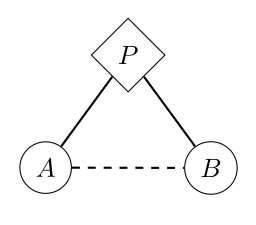This gives conceal and reveal operations (solid edges) going up and down the graph with respect to the virtual party $$P$$, as well as send and recv operations (dashed edges) between the parties. It’s worth noting here that $$A$$ and $$B$$ must be allowed to communicate, meaning that the notion of “connectedness” is talking about the ability to conceal and reveal, not send and receive. More work may need to be done on this design to make this clear.

Constituents may make up multiple protocols at once, which may even implement conversions directly between each other. In the graph below, virtual party $$A$$ represents an arithmetic protocol, while virtual party $$Y$$ represents a Yao protocol.

let A = protocol!([A, B]);
let Y = protocol!([A, B]);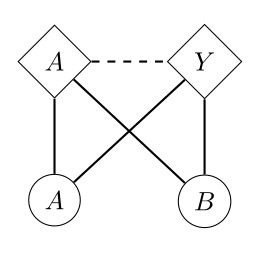In addition to parties participating in multiple protocols, the protocols themselves may participate in other protocols. This leads to “MPC in the head” style protocols.

let P1 = protocol!([A, B]);
let P2 = protocol!([A, B]);
let PP = protocol!([P1, P2]);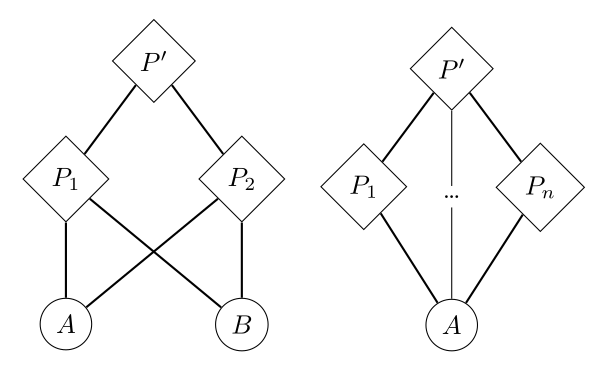Finally, we can construct protocols for which each layer is a recursive application of a protocol through some form of party-polymorphism.

let P<X, Y, Z> = protocol!([X, Y, Z]);
let P1 = P<A, B, C>;
let P2 = P<D, E, F>;
let P3 = P<G, H, I>;
let PH = P<P1, P2, P3>;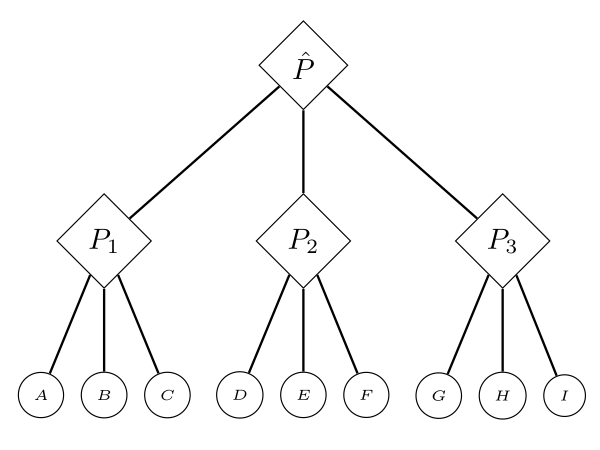fn calc3<@P>(a: bool@P, b: bool@P, c: bool@P);

// Works on a single layer of protocols.
let a = conceal!(@P1 <= @A { true });
let b = conceal!(@P1 <= @B { false });
let c = conceal!(@P1 <= @C { false });
calc3(a, b, c);

let d = conceal!(@P2 <= @D { false });
let e = conceal!(@P2 <= @E { true });
let f = conceal!(@P2 <= @F { true });
let g = conceal!(@P3 <= @G { true });
let h = conceal!(@P3 <= @H { false });
let i = conceal!(@P3 <= @I { true });

// Also works when we lift the values up to a higher protocol.
let abc = conceal!(@PH <= @P1 { a + b + c });
let def = conceal!(@PH <= @P2 { d + e + f });
let ghi = conceal!(@PH <= @P3 { g + h + i });
calc3(abc, def, ghi);


The details of this however, are still a work in progress.